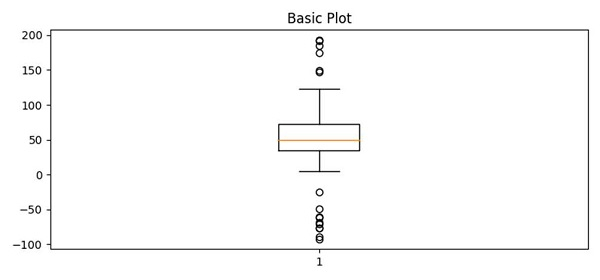# Pythonic way of detecting outliers in one dimensional observation data using Matplotlib

To detect outliers in one dimensional observation data, we can take the following Steps −

• Create spread, center, flier_high and flier_low.

• Using the above data (Step 1), we can calculate data.

• Use the suplots() method to create a figure and a set of subplots, i.e., fig1 and ax1.

• Set the title of ax1.

• Now using the boxplot() method and data, make a box and a whisker plot. Beyond the whiskers, data are considered outliers and are plotted as individual points.

• To display the figure, use the show() method.

## Example

from matplotlib import pyplot as plt
import numpy as np
plt.rcParams["figure.figsize"] = [7.50, 3.50]
plt.rcParams["figure.autolayout"] = True
np.random.seed(19680801)
center = np.ones(25) * 50
flier_high = np.random.rand(10) * 100 + 100
flier_low = np.random.rand(10) * -100
data = np.concatenate((spread, center, flier_high, flier_low))
fig1, ax1 = plt.subplots()
ax1.set_title('Basic Plot')
ax1.boxplot(data)
plt.show()

## OutputUpdated on: 09-Apr-2021

69 Views